# Kinematics in One Dimension

## Introduction

Motion: change in position or orientation with respect to time.

### Different Types of Motion We'll look at:... or a combination of them.

### One dimensional kinematics

For the case of 1-dimensional motion, we'll only consider a change of position in one direction.

It could be any of the three coordinate axes.

Just a description of the motion, without attempting to analyze the cause. To describe motion we need:

1. Coordinate System (origin, orientation, scale)
2. the object which is moving

### Particle model

We'll need to use an abstraction:

All real world objects take up space. We'll assume that they don't. In other words, things like cars, cats, and ducks are just point-like particles.

## Displacement Vector

To quantify the motion, we'll start by defining the displacement vector.

$$\Delta \mathbf{x} = \mathbf{x} - \mathbf{x}_0$$

In the case of our wandering bug, this would be the difference between the final position and the initial position.

#### Note on Notation!

$x_f$ is the same thing as $x$

$x_i$ is the same thing as $x_0$

### Displacement in 1-DHere's a car that moves from $\mathbf{x}_0$ to $\mathbf{x}$ creating a displacement vector of:

$$\Delta \mathbf{x} = \mathbf{x} - \mathbf{x}_0 = 60 \textrm{ m} - 0 \textrm{ m} = 60 \textrm{ m}$$

The car then reverses to $x = -20$.The leads to a displacement vector of $\Delta \mathbf{x} = -80 \textrm{m}$.

### Distance Traveled

To get the distance traveled, we just neet to take the magnitude of the displacement during a certain motion.

$$|\Delta \mathbf{x}| = \textrm{Distance Traveled}$$

## Speed and Velocity

$$\bbox[10px,border:3px solid green]{\textrm{Average Speed} \equiv \frac{\textrm{Distance in a given time}}{\textrm{Elapsed time}}}$$

The 'elapsed time' is determined in the same way as the distance: $\Delta t = t - t_0.$

Again, $t_0$ is the starting time, and $t$ is the final time.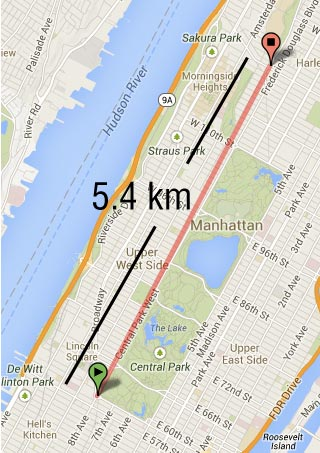Taking the A train between 59th and 125th takes about 8 minutes. The C, which is a local, takes 12 minutes (on a good day). Find the average speed for both of these trips.

### ...with a direction

Calculating the average speed didn't tell us anything about the direction of travel. For this, we'll need average velocity.

$$\bbox[10px,border:2px solid green]{\textrm{Average Velocity} \equiv \frac{\textrm{Displacement}}{\textrm{Elapsed time}}}$$

In mathematical terms:

$$\bbox[10px,border:2px solid red]{\overline{\mathbf{v}} \equiv \frac{\mathbf{x} - \mathbf{x}_0}{t-t_0} = \frac{\Delta \mathbf{x}}{\Delta t} }$$
(SI units of average velocity are m/s)This is a graph showing the position of an object with respect to time. Which choice best describes this motion?

1. The object is moving at 0.5 m/s in the +x direction.
2. The object is moving at 1.0 m/s in the +x direction.
3. The object is moving at 2.0 m/s in the +x direction.
4. The object is not moving at all.This is a graph showing the position of an object with respect to time. Which choice best describes this motion?

1. The object is moving at 0.5 m/s in the +x direction.
2. The object is moving at 1.0 m/s in the +x direction.
3. The object is moving at 2.0 m/s in the +x direction.
4. The object is not moving at all.

Thinking about the A train, it's clear that its speed and velocity stayed essentially constant between 59th and 125th ideally). However, the C train had to start and stop at 7 stations. To quantify, this difference in motion, we'll need to introduce the concept of instantaneous velocity.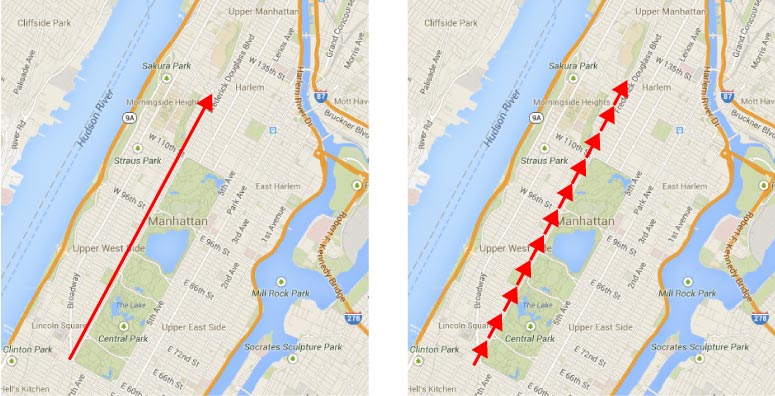If we imagine making many measurements of the velocity over the course of the travel, by reducing the $\Delta x$ we are considering, then we can begin to see how we can more accurately assess the motion of the train.

The concept of instantaneous velocity involves considering an infinitesimally small section of the motion:

$$\mathbf{v} = \lim_{\Delta t \rightarrow 0} \frac{\Delta \mathbf{x}}{\Delta t} = \frac{dx}{dt}$$

This will enable us to talk about the velocity at a particle's specific position or time rather than for an entire trip.

In general, this is what we'll mean when we say 'velocity' or 'speed'.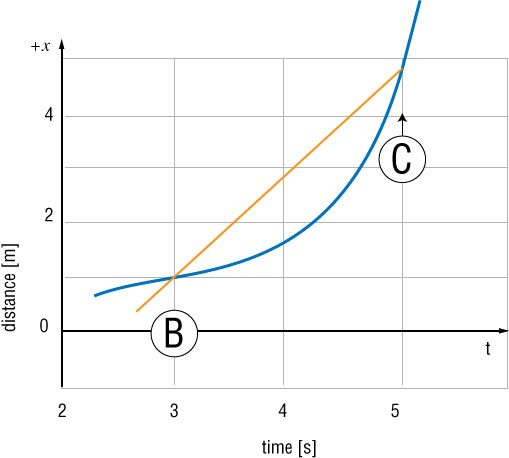At which of the following times is the speed of this object the greatest?

1. $t = 0$
2. $t = 2$ s
3. $t = 4$ s
4. $t = 8$ s

## Change in velocity.

Naturally, in order to begin moving, an object must change its velocity.

Here's a graph of a bicyclist riding at a constant velocity. (In this case it's 10 m/s)Now, here's a graph of the same bicyclist riding and changing his velocity during the motion### Acceleration

This change in velocity we'll call acceleration, and we can define it in a very similar way to our definition of velocity:

$$\overline{\mathbf{a}} = \frac{\mathbf{v}-\mathbf{v}_0}{t-t_0} = \frac{\Delta \mathbf{v}}{\Delta t}$$

Again, in this case we're talking about average acceleration.

At $t=0$, the A train is at rest at 59th street. 5 seconds later, it's traveling north at 19 meters per second. What is the average acceleration during this time interval?

If we considered the same very small change in time, the infinitesimal change, then we could talk about instantaneous acceleration

$$\mathbf{a} = \lim_{\Delta t \rightarrow 0} \frac{\Delta \mathbf{v}}{\Delta t} = \frac{dv}{dt}$$

The SI units of acceleration are meters per second per second, or $m s^{-2}$. That's probably a little bit of a weird unit, but, it makes sense to think about like this:

$$\frac{\left(\frac{m}{s}\right)}{s} \textrm{ or } \frac{vel}{s}$$This is a graph showing the velocity of an object with respect to time. Which choice best describes this motion?

1. The object is moving at the same velocity, which is 3 m/s.
2. The object starts at rest, and increases its velocity, for ever.
3. The object starts at rest, then increases its velocity for a while, then stops moving after 3 seconds.
4. The object starts at rest, then increases its velocity, then moves at the same speed after t = 3s.

### Acceleration, the math.

To quantify to the acceleration of a moving body, say this car, we'll need to know its initial and final velocities

The car has a build in speedometer, so we can look at that to get the speed, and if we don't change direction, then the velocity will be always pointed in the same direction.

For this case of a car starting from rest, and then increasing velocity, the acceleration will be a positive quantity.

$$\mathbf{\overline{a}} = \frac{\mathbf{v} - \mathbf{v}_0}{t-t_0} = \frac{20 \textrm{mph} - 0 \textrm{mph}}{2 \rm s - 0 s} = \frac{20 \textrm{mph}}{2 \rm s}$$

$$\mathbf{\overline{a}} = \frac{9 \rm m/s}{2 \rm s} = + 4.5 \rm ms^{-2}$$

### Slowing down

What if we ask about a car slowing down. Now, our $\mathbf{v}_0 = +9m/s$ while $\mathbf{v} = 0$.

Now the math looks like this:

$$\mathbf{\overline{a}} = \frac{\mathbf{v} - \mathbf{v}_0}{t-t_0} = \frac{0 \textrm{m/s} - 9 \textrm{m/s}}{2 \rm s - 0 s} = -\frac{9 \textrm{m/s}}{2 \rm s} = -4.5 \rm m/s^2$$

We notice that the acceleration is negative.

### Acceleration in the negative

What if the car starts accelerating in the negative direction?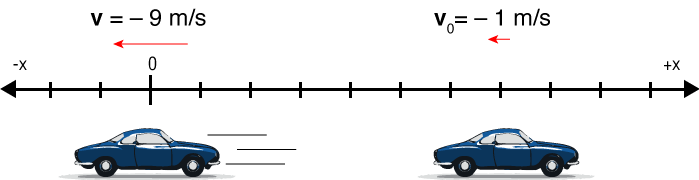Now, even the speed is increasing, the velocity is getting more negative.

If we do the math, we'll see that the acceleration vector points in the negative direction.

### Summary of acceleration signage.

When the signs of an object’s velocity and acceleration are the same (in same direction), the object is speeding up
When the signs of an object’s velocity and acceleration are opposite (in opposite directions), the object is slowing down and speed decreasesAt one particular moment, a subway train is moving with a positive velocity and negative acceleration. Which of the following phrases best describes the motion of this train? Assume the front of the train is pointing in the positive x direction.

1. The train is moving forward as it slows down.
2. The train is moving in reverse as it slows down.
3. The train is moving faster as it moves forward.
4. The train is moving faster as it moves in reverse.
5. There is no way to determine whether the train is moving forward or in reverse.

At one particular moment, a subway train is moving with a negative velocity and positive acceleration. Which of the following phrases best describes the motion of this train? Assume the front of the train is pointing in the positive x direction.

1. The train is moving forward as it slows down.
2. The train is moving in reverse as it slows down.
3. The train is moving faster as it moves forward.
4. The train is moving faster as it moves in reverse.
5. There is no way to determine whether the train is moving forward or in reverse.

A car is moving in the negative direction but slowing down. What is the sign of the car's acceleration?

1. Positive
2. Negative
3. Acceleration is equal to 0.What is the average velocity of this object between 0 and 3 seconds?

1. 3 m/s
2. 4 m/s
3. 6 m/s
4. 12 m/s

Question 1: Someone is walking

## Kinematic equations

1. $$\overline{a} = a = \frac{v-v_0}{t} \;\; \Rightarrow \;\; v = v_0 + at$$
2. $$\overline{v} = \frac{x-x_0}{t-t_0} \;\; \Rightarrow \;\; x-x_0 = \overline{v}t = \frac{1}{2}(v_0+v)t$$
3. We can do a lot by rearranging these equations.

Putting $v$ from (1) into (2) will give us:

4. $$x-x_0 = v_0 t + \frac{1}{2}a t^2$$
5. or, solving (1) for $t$, then inserting that into (2) will give us:

6. $$v^2 = v_0^2 + 2a(x-x_0)$$
1. $v = v_0 + at$
2. $x = \overline{v}t = \frac{1}{2}(v_0+v)t$
3. $x = x_0 + v_0 t + \frac{1}{2}a t^2$
4. $v^2 = v_0^2 + 2ax$
$$\bbox[10px,border:2px solid red]{v = v_0 + at}$$

Here we have an equation for velocity which is changing due to an acceleration, $a$.

It tells us how fast something will be going (and the direction) if has been accelerated for a time, $t$.

• It can determine an object’s velocity at any time t when we know its initial velocity and its acceleration
• Does not require or give any information about position
• Ex: “How fast was the car going after 10 seconds while accelerating from rest at 10 m/s$^2$”
• Ex: “How long did it take to reach 20 miles per hour”
$$\bbox[10px,border:2px solid red]{x = \bar{v}t = \frac{(v + v_0)t}{2}}$$

This equation will tell us the position of an object based on the initial and final velocities, and the time elapsed.

It does not require knowing, nor will it give you, the acceleration of the object.

• Ex: How far did the duck walk if it took 10 seconds to reach 50 miles per hour under constant acceleration.
$$\bbox[10px,border:2px solid red]{x = x_0 + v_0 t + \frac{a t^2}{2}}$$

Gives position at time t in terms of initial velocity and acceleration

• Doesn’t require or give final velocity.
• Ex: “How far up did the rocket go?”
$$\bbox[10px,border:2px solid red]{v^2 = v_0^2 + 2ax}$$

Gives velocity at time t in terms of acceleration and position

• Does not require or give any information about the time.
• Ex: “How fast was penny going when it reached the bottom of the well?”

### Equations of Motion (1-D)

Things to be aware of:

1. They are only for situations where the acceleration is constant.
2. The way we have written them is really just for 1-D motion.
Equation Missing Variable Good for finding
$v = v_0 + at$ $x$ $a$,$t$,$v$
$x = \frac{(v + v_0)t}{2}$ $a$ $x$,$t$,$v$
$x = x_0+ v_0 t + \frac{a t^2}{2}$ $v$ $x$,$a$,$t$
$v^2 = v_0^2 + 2ax$ $t$ $a$,$x$,$v$

## Solving Problems

1. Diagram: draw a picture
2. Characters: Consider the problem a story. Who are the characters?
3. Find: clearly list symbolically what we're looking for.
4. Solve: state the basic idea behind solution, in a few words (physical principles used, etc.)
5. Assess: does answer make sense?

A taxi is sitting at a red light. The light turns green and the taxi accelerates at 2.5 m/s$^2$ for 3 seconds. How far does it travel during this time?

A particle is at rest. What acceleration value should we give it so that it will be 2 meters away from its starting position after 0.4 seconds?

A subway train accelerates starting at x = 200 m uniformly until it reaches x = 350 m, at a uniform acceleration value of 0.5 m/s$^2$.

1. If it had an initial velocity of 0 m/s, what will the duration of this acceleration be?
2. If it had an initial velocity of 8 m/s, what will the duration of this acceleration be?

If $x(t) = 4-27t +t^3$, find $v(t)$ and $a(t)$. Also, find the time when the velocity is zero.Traian Vuia, a Romanian Inventor, wanted to reach 17 m/s in order to take off in his flying machine. His plane could accelerate at 2m/s$^2$. The only runway he had access to was 80 meters long. Will he reach the necessary speed?

## PlottingLet's look at the motion of a honey badger.

After each second, we note where the honey badger is along the x axis.

t[s] x[ft]
0 0.00
1 5.00
2 10.0
3 15.0
4 17.5
5 20.0
6 22.5
7 25.0
8 35.0
9 50.0### Plots -> Equations

 $$\mathbf{v} = \lim_{\Delta t \rightarrow 0} \frac{\Delta \mathbf{x}}{\Delta t} = \frac{dx}{dt}$$ Velocity is the slope of position (w.r.t time) $$\mathbf{a} = \lim_{\Delta t \rightarrow 0} \frac{\Delta \mathbf{v}}{\Delta t} = \frac{dv}{dt}$$ Acceleration is the slope of velocity (w.r.t time)

The first plot shows the position vs. time graph for an object in motion. Which of the velocity vs. time graphs shown would best correspond to this motion?Which of the following velocity vs. time graphs represents an object with a negative constant acceleration?Derive kinematics using calculus.$$v = v_0 + at$$ $$x = vt$$ $$x = v_0 t + \frac{a t^2}{2}$$ velocity as a function of time: $v(t)$ Acceleration is constant position as a function of time $x(t)$. (vel. constant, accel = 0) position as a function of time

A car and a motorcycle are at $x_0 = 0$ at $t = 0$. The car moves at a constant velocity $v_0$. The motorcycle starts at rest and accelerates with constant acceleration a.

1. Find the $t$ where they meet.
2. Find the position $x$ where they meet.
3. Find the velocity of the motorcycle when they meet.

Question 4: An object in motion

## Free Fall

A freely falling object is any object moving freely under the influence of gravity alone.

Object could be:

1. Dropped = released from rest
2. Thrown downward
3. Thrown upward

It does not depend upon the initial motion of the object.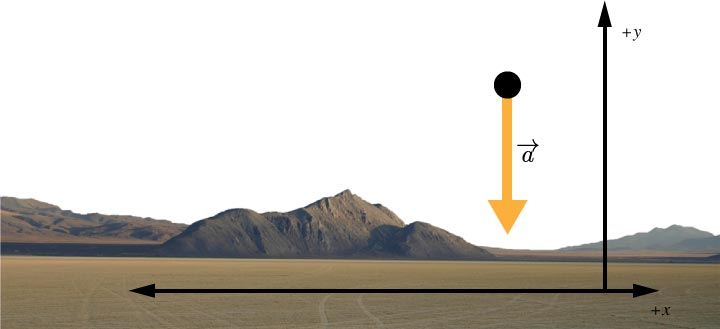1. The acceleration of an object in free fall is directed downward (negative direction), regardless of the initial motion.
2. The magnitude of free fall acceleration is $\bbox[5px,border:2px solid red]{9.8 \textrm{m}/\textrm{s}^2 = g}$.
3. We can neglect air resistance.
4. We'll choose our y axis to be positive upward.
5. Consider motion near Earth’s surface for now.
bowling ball and a feather

Kinematic equation in the case of free fall:

1. $v = v_0 - gt$
2. $y = \overline{v}t = \frac{1}{2}(v_0+v)t$
3. $y = y_0 + v_0 t - \frac{1}{2}g t^2$
4. $v^2 = v_0^2 - 2gy$

They are the same. We just replaced $x \rightarrow y$ and $a \rightarrow -g$.

An object is thrown upward at 20 m/s:

1. How long will it take to reach the top
2. How high is the top?
3. How long to reach the bottom?
4. How fast will it be going when it reaches the bottom?

An arrow is launched vertically upward. It moves straight up to a maximum height, then falls to the ground. Which graph best represents the vertical velocity of the arrow as a function of time? Ignore air resistance; the arrow is in free fall!.If an object is thrown upward from a height $y_0$ with a speed $v_0$, when will it hit the ground?

### Drop a wrench

A worker drops a wrench down the elevator shaft of a tall building.

1. Where is the wrench 1.5 seconds later?
2. How fast is the wrench falling at that time?

A rock is thrown upward with a velocity of 49 m/s from a point 15 m above the ground.

1. When does the rock reach its maximum height?
2. What is the maximum height reached?
3. When does the rock hit the ground?